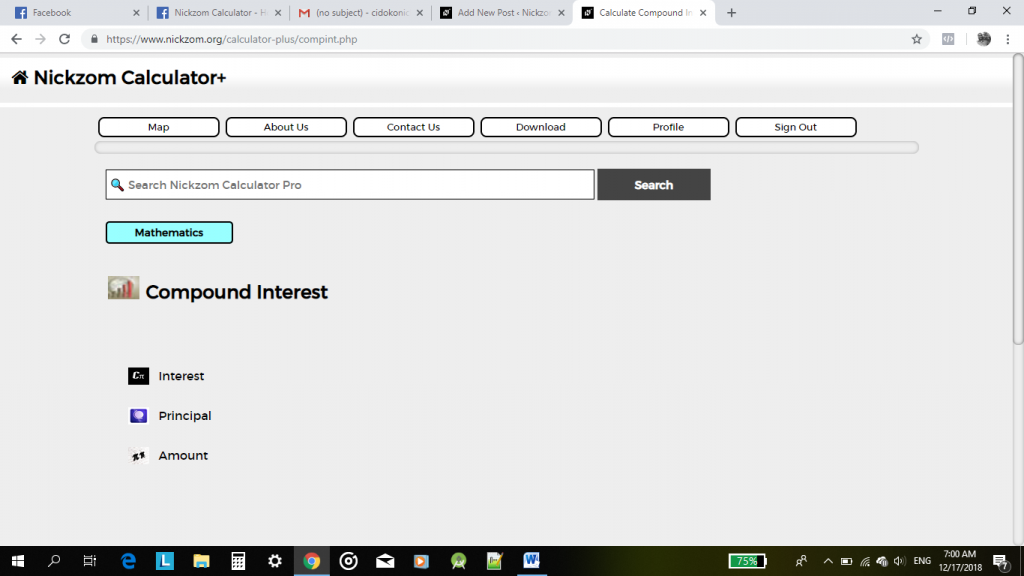## Calculating Compound Interest Using Nickzom Calculator

Using the Nickzom Calculator for Math calculations is quite simple. Navigation through any of the App platforms from Mobile to Web is quite easy. Just a bit of playing around and you’re quite comfortable with the user interface. Does Math seem hard, Nickzom Calculator makes it easier.

We will consider Compound Interest, how your Principal, Interest and Amount can easily be calculated using the Nickzom calculator. The platform  also goes a long way in showing the formula used, and the steps it took to arrive at its answer.Compound Interest

Compound Interest being a very popular topic in Mathematics entails basically  Principal, Rate, Interest, Time (number of years) and expected amount. The Nickzom Calculator can carry out calculations on Compound Interest, solving problems on